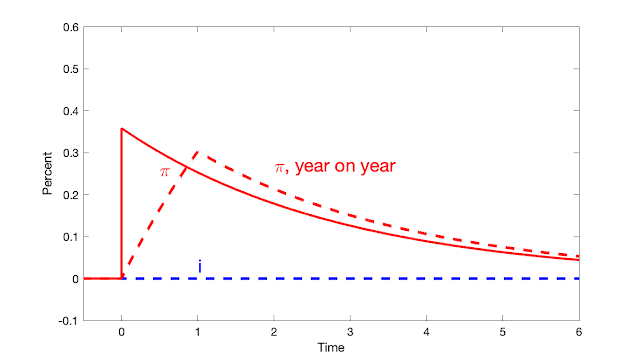## Rabu, 05 Juli 2023

### Fiscal inflation and interest rates

Economics is about solving lots of little puzzles. At a July 4th party, a super smart friend -- not a macroeconomist -- posed a puzzle I should have understood long ago, prompting me to understand my own models a little better.

How do we get inflation from the big fiscal stimulus of 2020-2021, he asked? Well, I answer, people get a lot of government debt and money, which they don't think will be paid back via higher future taxes or lower future spending. They know inflation or default will happen sooner or later, so they try to get rid of the debt now while they can rather than save it. But all we can do collectively is to try to buy things, sending up the price level, until the debt is devalued to what we expect the government can and will pay.

OK, asked my friend, but that should send interest rates up, bond prices down, no? And interest rates stayed low throughout, until the Fed started raising them. I mumbled some excuse about interest rates never being very good at forecasting inflation, or something about risk premiums, but that's clearly unsatisfactory.

Of course, the answer is that interest rates do not need to move. The Fed controls the nominal interest rate. If the Fed keeps the short term nominal interest rate constant, then nominal yields of all bonds stay the same, while fiscal inflation washes away the value of debt. I should have remembered my own central graph:

This is the response of the standard sticky price model to a fiscal shock -- a 1% deficit that is not repaid by future surpluses -- while the Fed keeps interest rates constant. The solid line is instantaneous inflation, while the dashed line gives inflation measured as percent change from a year ago, which is the common way to measure it in the data.

There you have it: The fiscal shock causes inflation, but since the nominal interest rate is fixed by the Fed, it goes nowhere, and long term bonds (in this linear model with the expectations hypothesis) go nowhere too.

OK for the result, but how does it work? What about the intuition, that seeing inflation coming we should see higher interest rates? Let's dig deeper.

Start with the simplest model, one-period debt and flexible prices. Now the model comes down to, nominal debt / price level = present value of surpluses, $\frac{B_{t-1}}{P_t} = E_t \sum_{j=0}^\infty \beta^j s_{t+j}.$ (If you don't like equations, just read the words. They will do.) With a decline in the present value of surpluses, the value of debt coming due today (top left) can't change, so the price level must rise. The price of debt coming due is fixed at 1, so its relative price can't fall and its interest rate can't rise. Or, this model describes a price level jump. We get bad fiscal news, people try to spend their bonds, the price level jumps unexpectedly up, ($$P_t$$ jumps up relative to $$E_{t-1}P_t$$, but there is no further inflation, no rise in expected inflation so the interest rate $$i_t = r+ E_t \pi_{t+1}$$ doesn't change.

Ok, fine, you say, but that's one period, overnight debt, reserves at the Fed only. What about long term bonds? When we try to sell them, their prices can go down and interest rates go up, no? No, because if the Fed holds the nominal interest rate constant, their nominal prices don't change. With long term bonds, the basic equation becomes market value of nominal debt / price level = expected value of surpluses, $\frac{\sum_{j=0}^\infty Q_t^{(j)} B_{t-1}^{(j)}}{P_t} = E_t \sum_{j=0}^\infty \beta^j s_{t+j}.$ Here, $$Q_t^{(j)}$$ is the price of $$j$$ period debt at time $$t$$, and $$B_{t-1}^{(j)}$$ is the face value of debt at the beginning of time $$t$$ that matures in time $$t+j$$. ($$Q_t^{(j)}=1/[1+y^{(j)}_t)]^j$$ where $$y^{(j)}_t$$ is the yield on $$j$$ period debt; when the price goes down the yield or long-term interest rate goes up. )

So, my smart friend notices, when the present value of surpluses declines, we could see nominal bond prices $$Q$$ on top fall rather than the price level $$P$$ on the bottom rise.  But we don't, because again, the Fed in this conceptual exercise keeps the nominal interest rate fixed, and so long term bond prices don't fall. If the $$Q$$ don't fall, the $$P$$ must rise.

The one-period price level jump is not realistic, and the above graph plots what happens with sticky prices. (This is the standard continuous time new-Keynesian model.) The intuition is the same, but drawn out. The sum of future surpluses has fallen. People try to sell bonds, but with a constant interest rate the nominal price of long term bonds cannot fall. So, they try to sell bonds of all maturities, pushing up the price of goods and services. With sticky prices, this takes time; the price level slowly rises as inflation exceeds the nominal interest rate. A drawn out period of low real interest rates slowly saps the value of bondholder's wealth. In present value terms, the decline in surpluses is initially matched by a low real discount rate. Yes, there is expected inflation. Yes, long-term bondholders would like to escape it. But there is no escape: real rates of return are low on all bonds, short-term and long term.

So, dear friend, we really can have a period of fiscal inflation, with no change in nominal interest rates. Note also that the inflation eventually goes away, so long as there are no more fiscal shocks, even without the Fed raising rates. That too seems a bit like our reality. This has all been in my own papers for 20 years. It's interesting how hard it can be to apply one's own models right on the spot. Maybe it was the great drinks and ribs.